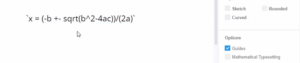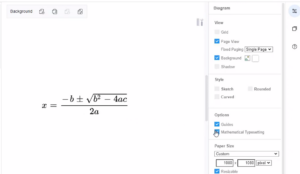In VP Online, you can enter text in LaTeX, or AsciiMath format, then click on the diagram and select Mathematical typesetting, to turn it into a mathematical formula. In this video, you can see how to write mathematical typesetting in design.

1. First, enter your maths equation using LaTeX or AsciiMath in a text shape.

• AsciiMath between , for example: a^2+b^2 = c^2`
• LaTeX between $$, for example:$$\sqrt{3×-1}+(1+x)^2
• Inline LaTeX between $$and$$, for example: $$\sqrt{3×-1}+(1+x)^2$$Tips: You can mix inline LaTeX and AsciiMath in the same text2. After then, you can enable mathematical typesetting by clicking “Mathematical Typesetting” in the Diagram options.Enabling mathematical typesetting will use MathJax to render your maths equations. MathJax renders equations neatly and works in all browsers.